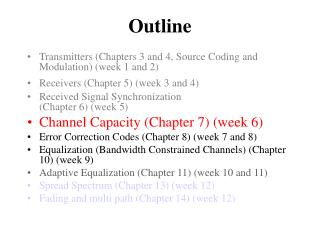DownloadDownload PresentationOutline

# Outline

Télécharger la présentation## Outline

- - - - - - - - - - - - - - - - - - - - - - - - - - - E N D - - - - - - - - - - - - - - - - - - - - - - - - - - -
##### Presentation Transcript

1. Outline • Transmitters (Chapters 3 and 4, Source Coding and Modulation) (week 1 and 2) • Receivers (Chapter 5) (week 3 and 4) • Received Signal Synchronization (Chapter 6) (week 5) • Channel Capacity (Chapter 7) (week 6) • Error Correction Codes (Chapter 8) (week 7 and 8) • Equalization (Bandwidth Constrained Channels) (Chapter 10) (week 9) • Adaptive Equalization (Chapter 11) (week 10 and 11) • Spread Spectrum (Chapter 13) (week 12) • Fading and multi path (Chapter 14) (week 12)

2. Channel Capacity (Chapter 7) (week 6) • Discrete Memoryless Channels • Random Codes • Block Codes • Trellis Codes

3. Channel Models • Discrete Memoryless Channel • Discrete-discrete • Binary channel, M-ary channel • Discrete-continuous • M-ary channel with soft-decision (analog) • Continuous-continuous • Modulated waveform channels (QAM)

4. Discrete Memoryless Channel • Discrete-discrete • Binary channel, M-ary channel Probability transition matrix

5. Discrete Memoryless Channel • Discrete-continuous • M-ary channel with soft-decision (analog) output x0 x1 x2 . . . xq-1 AWGN y

6. Discrete Memoryless Channel • Continuous-continuous • Modulated waveform channels (QAM) • Assume Band limited waveforms, bandwidth = W • Sampling at Nyquist = 2W sample/s • Then over interval of N = 2WT samples use an orthogonal function expansion:

7. Discrete Memoryless Channel • Continuous-continuous • Using orthogonal function expansion:

8. Discrete Memoryless Channel • Continuous-continuous • Using orthogonal function expansion get an equivalent discrete time channel: Gaussian noise

9. Capacity of binary symmetric channel • Average Mutual Information

10. Capacity of binary symmetric channel • Channel Capacity is Maximum Information • earlier showed:

11. Capacity of binary symmetric channel • Channel Capacity • When p=1 bits are inverted but information is perfect if invert them back!

12. Capacity of binary symmetric channel • Effect of SNR on Capacity • Binary PAM signal (digital signal amplitude 2A) AGWN

13. Capacity of binary symmetric channel • Effect of SNR • Binary PAM signal (digital signal amplitude 2A)

14. Capacity of binary symmetric channel • Effect of SNR • Binary PAM signal (digital signal amplitude 2A)

15. Capacity of binary symmetric channel • Effect of SNR • Binary PAM signal (digital signal amplitude 2A) Not sure about this Does it depend on bandwidth?

16. Capacity of binary symmetric channel • Effect of SNR • Binary PAM signal (digital signal amplitude 2A)

17. Capacity of binary symmetric channel • Effect of SNR • Binary PAM signal (digital signal amplitude 2A)

18. Capacity of binary symmetric channel • Effect of SNR • Binary PAM signal (digital signal amplitude 2A) At capacity SNR = 7, so waste lots of SNR to get low BER!!!

19. Capacity of binary symmetric channel • Effect of SNRb • Binary PAM signal (digital signal amplitude 2A)

20. Channel Capacity of Discrete Memoryless Channel • Discrete-discrete • Binary channel, M-ary channel Probability transition matrix

21. Channel Capacity of Discrete Memoryless Channel Average Mutual Information

22. Channel Capacity of Discrete Memoryless Channel Channel Capacity is Maximum Information Occurs for only if Otherwise must work out max

23. x0 x1 x2 . . . xq-1 y Channel Capacity Discrete Memoryless Channel • Discrete-continuous • Channel Capacity

24. x0 x1 x2 . . . xq-1 y Channel Capacity Discrete Memoryless Channel • Discrete-continuous • Channel Capacity with AWGN

25. x0 x1 x2 . . . xq-1 y Channel Capacity Discrete Memoryless Channel • Binary Symmetric PAM-continuous • Maximum Information when:

26. Channel Capacity Discrete Memoryless Channel • Binary Symmetric PAM-continuous • Maximum Information when:

27. Channel Capacity Discrete Memoryless Channel • Binary Symmetric PAM-continuous • Versus Binary Symmetric discrete

28. Discrete Memoryless Channel • Continuous-continuous • Modulated waveform channels (QAM) • Assume Band limited waveforms, bandwidth = W • Sampling at Nyquist = 2W sample/s • Then over interval of N = 2WT samples use an orthogonal function expansion:

29. Discrete Memoryless Channel • Continuous-continuous • Using orthogonal function expansion get an equivalent discrete time channel: Gaussian noise

30. Discrete Memoryless Channel • Continuous-continuous • Capacity is (Shannon)

31. Discrete Memoryless Channel • Continuous-continuous • Maximum Information when: Statistically independent zero mean Gaussian inputs then

32. Discrete Memoryless Channel • Continuous-continuous • Constrain average power in x(t):

33. Discrete Memoryless Channel • Continuous-continuous • Thus Capacity is:

34. Discrete Memoryless Channel • Continuous-continuous • Thus Normalized Capacity is: